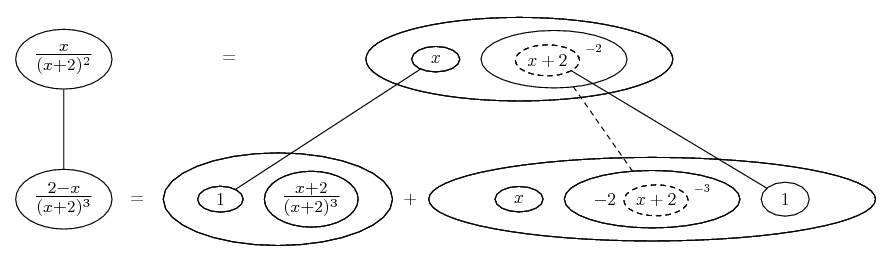# Thread: Clarification on the Quotient Rule

1. ## Clarification on the Quotient Rule

Hello, and thanks for your help in advance.

I recently learned the quotient rule in finding the derivative of more complicated expressions and had a question on it.

The way we've been taught is that when given f(x)/g(x), the derivative is [g(x)*f'(x)-f(x)*g'(x)]/g(x)^2

My question has to do with when the more complicated expression is in the denominator of the function we are to differentiate. A good example is stated below:

....x.....
(x+2)^2

(The periods are present just to make the fraction look better.)

In this situation, the denominator of the derivative gets very complicated if I square [(x+2)^2], so is it legitimate to switch which expression is f(x) and g(x), making the derivative have x^2 in the denominator and changing the numerator accordingly?

I realize my question is a bit confusing, so please don't hesitate to ask questions...just hoping to keep expressions as simplified as possible.

2.Originally Posted by NBrunkHello, and thanks for your help in advance.

I recently learned the quotient rule in finding the derivative of more complicated expressions and had a question on it.

The way we've been taught is that when given f(x)/g(x), the derivative is [g(x)*f'(x)-f(x)*g'(x)]/g(x)^2

My question has to do with when the more complicated expression is in the denominator of the function we are to differentiate. A good example is stated below:

....x.....
(x+2)^2

(The periods are present just to make the fraction look better.)

In this situation, the denominator of the derivative gets very complicated if I square [(x+2)^2], so is it legitimate to switch which expression is f(x) and g(x), making the derivative have x^2 in the denominator and changing the numerator accordingly?

I realize my question is a bit confusing, so please don't hesitate to ask questions...just hoping to keep expressions as simplified as possible.
how more complicated? ... squaring $\displaystyle (x+2)^2$ results in $\displaystyle (x+2)^4$ ... you are not required to expand the result.

3. No, sorry! The different things done to f and g depend exactly on their being numerator and denominator respectively. However, you don't have to square the bottom, you can just raise the power by one... will edit in a pic in a couple of mins... where...... is the chain rule, here wrapped inside the legs-uncrossed version of...... the product rule. Straight continuous lines differentiate downwards (integrate up) with respect to x, and the straight dashed line similarly but with respect to the dashed balloon expression (the inner function of the composite which is subject to the chain rule).

Could have done without the chain rule shape in this case, as the inner function differentiates to 1. Anyway, this (which is essentially the same as Calculus26's below) is one way to approach quotients - as a product in which one part of the product is raised to a negative power.

__________________________________________

Don't integrate - balloontegrate!

http://www.ballooncalculus.org/examples/gallery.html

http://www.ballooncalculus.org/asy/doc.html

4. For x/(x+2)^2

Simply

f ' = [(x+2)^2 - x(2(x+2))]/ (x+2)^4

= [x+2 - 2x]/(x+2)^3

= (2-x)/(x+2)^3

You don't want to square out (x+2)

As to your more general question you could make the substitution

u = x+2

x= u-2

then you have

f(u) = (u-2)/u^2

then df/dx = df/du*du/dx = (u^2 - (u-2)2u)/u^4 *1

= [4-u]/u^3

=[2-x]/(x+2)^3 as before

#### Search Tags

clarification, quotient, quotient rule, rule